# Kinetic Energy of Gas Formula

Kinetic Energy of Gas Formula

In an ideal gas, there are no attractive forces between the gas molecules, and there is no rotation or vibration within the molecules. The kinetic energy of the translational motion of an ideal gas depends on its temperature. The formula for the kinetic energy of a gas defines the average kinetic energy per molecule. The kinetic energy is measured in Joules (J), and the temperature is measured in Kelvin (K).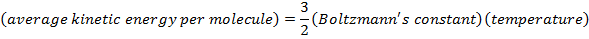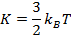K = average kinetic energy per molecule of gas (J)

kB = Boltzmann's constant (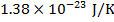)

T = temperature (k)

Kinetic Energy of Gas Formula Questions:

1) Standard Temperature is defined to be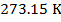. What is the average translational kinetic energy of a single molecule of an ideal gas at Standard Temperature?

Answer: The average translational kinetic energy of a molecule of an ideal gas can be found using the formula: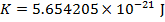The average translational kinetic energy of a single molecule of an ideal gas is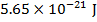(Joules).

2) One mole (mol) of any substance consists of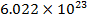molecules (Avogadro's number). What is the translational kinetic energy of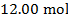of an ideal gas at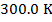?

Answer: The translational kinetic energy ofof an ideal gas can be found by multiplying the formula for the average translational kinetic energy by the number of molecules in the sample. The number of molecules is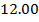times Avogadro's number: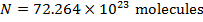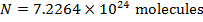The translational kinetic energy for theof ideal gas atis: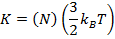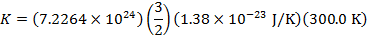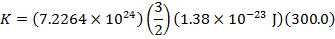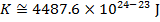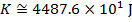The translational kinetic energy ofof ideal gas atis(Joules).